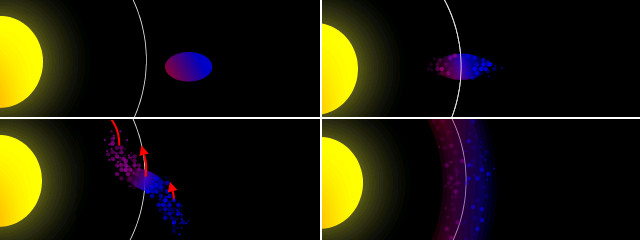# The Difference Between Centre of Mass and Centre of Gravity

Many people assume that the terms “centre of mass” and “centre of gravity” are synonymous, but this is not the case.

Centre of mass is the point at which the distribution of mass is equal in all directions, and does not depend on gravitational field. Centre of gravity is the point at which the distribution of weight is equal in all directions, and does depend on gravitational field.A toy bird balances when a pivot is placed at its centre of gravity.

The centre of mass and the centre of gravity of an object are in the same position if the gravitational field in which the object exists is uniform. In most cases this is true to a very good approximation: even at the top of Mount Everest (8848 metres) the gravitational field strength is still 99.6% of its standard value. You are unlikely ever to experience a difference between centre of mass and centre of gravity, as the gravitational field in which you find yourself is extremely uniform.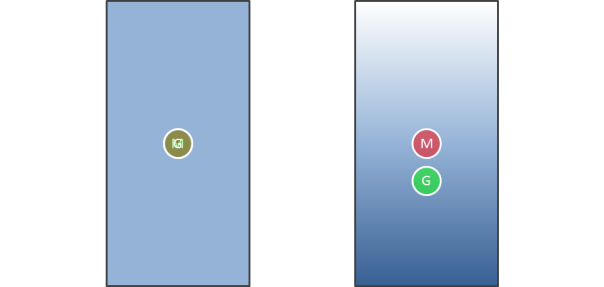The object on the left, in a uniform gravitational field, has overlapping centres of gravity and mass. For the object on the right, in which the gravitational field is stronger towards its base, the centre of gravity is below the centre of mass. Approaching a black hole the gradient of the gravitational field would be infinitely “steep”, leading to an incredible difference in gravitational field and death by spaghettification for anyone falling into a black hole.

# Hohmann Transfers

The Hohmann transfer is an orbital manoeuvre used to transfer a satellite between two different circular orbits.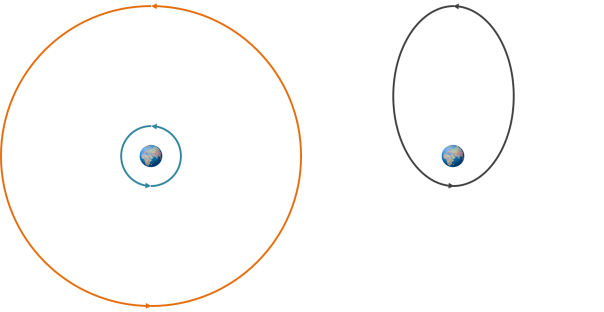On the left, the two circular orbits between which the transfer will take place. On the right, the elliptical Hohmann transfer orbit.

The orbit of the lower (blue) orbit has the lowest energy (i.e. the specific orbital energy) of the three, the Hohmann transfer orbit has a higher energy than that, and the highest (orange) orbit has the greatest energy of the three.  The gravitational potential and kinetic energies of the initial and final circular orbits are fairly constant, but the gravitational potential and kinetic energies of the Hohmann transfer orbit vary substantially as the orbiting object transfers gravitational potential to kinetic as it approaches Earth and vice versa.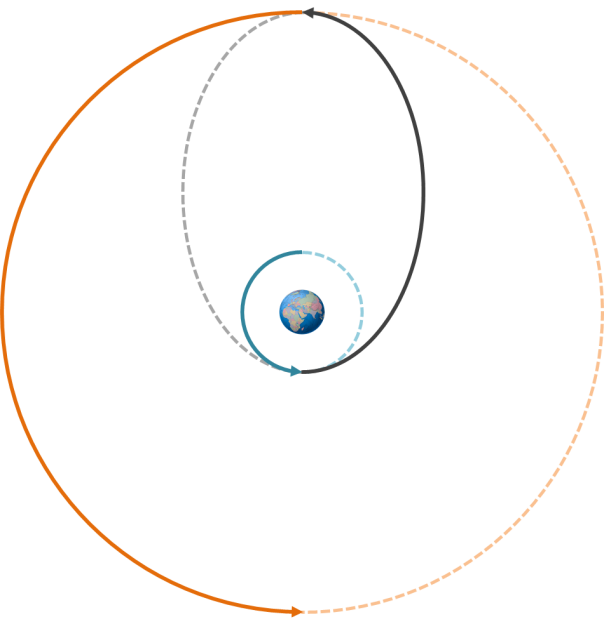The Hohmann transfer takes place along (half of) an elliptical orbit with one half of the ellipse touching the lower orbit and the other half touching the higher orbit. Two different thruster impulses are used: one to move it onto the elliptical orbit, and then a second one to move it onto the higher orbit. Each time the thruster is fired this increases the kinetic energy of the satellite, which is then transferred to the gravitational potential energy of its new orbit. Because orbits are reversible, moving from a higher orbit to a lower orbit still involves two impulses, but they are in a direction opposite to the motion of the satellite, causing it to decrease in speed and “fall” into the lower orbit.

# Real and Apparent Weightlessness

The strength of the gravitational field at Earth’s surface g is 9.81 newtons per kilogram. This means that every kilogram of mass feels a force of 9.81 newtons pulling it downwards towards the centre of Earth.

As you climb higher and higher, the value of g becomes smaller and smaller. At the peak of Mount Everest, g?=?9.79?N/kg, and at the summit of Chimborazo, the farthest point from Earth’s centre, g?=?9.78?N/kg.

So what is the value of g aboard the International Space Station, in orbit around Earth? You would be forgiven for thinking that the answer is 0?N/kg, because the environment the astronauts are in is often described as “zero-g”, but this is not the case. The value of g aboard the ISS is actually 8.65?N/kg, only 12% less than on Earth’s surface.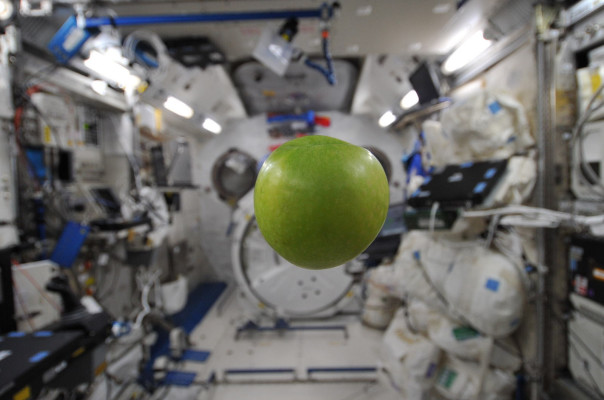An apple floats in mid-air aboard the ISS.

The astronauts aboard the ISS experience apparent weightlessness, not true weightlessness. The reason they appear to be in a zero-g environment is only because they are in orbit around the Earth – if the ISS were to slow to a halt it would fall towards Earth just like an object on Earth’s surface (though it’d fall a little bit slower at first).

In orbit the ISS is falling towards Earth at just the same rate as Earth is curving away, keeping it at a constant distance from Earth’s surface.* Because astronauts are in constant freefall they don’t push against the “floor” and the “floor” doesn’t push against them and therefore they feel weightless. If you’ve ever felt a little bit lighter in a lift as it started to move downwards, just imagine that effect taken to an extreme in a lift shaft where you can never hit the bottom.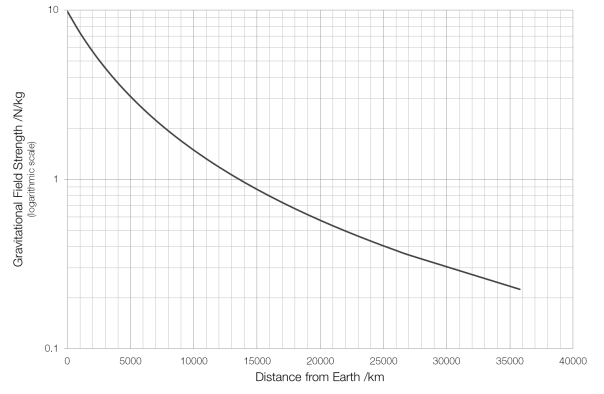Even geostationary satellites, like those that supply satellite TV, at a distance of 36000 km from Earth aren’t in zero-g. If they weren’t within Earth’s gravitational field they wouldn’t orbit and would fly off into space; for geostationary satellites g?=?0.224?N/kg.

The closest human beings have ever been to true zero-g is on the way to the Moon, at the point at which the gravitational pull of the Earth in one direction was equal to the gravitational pull of the Moon in the other direction. Because the mass of the Moon is about 1/81 of the mass of the Earth and the strength of a body’s gravitational field depends on the square of the distance from the body this point is about nine-tenths of the distance between the two: 346?000 km from Earth. At this point the pull from the Earth is 0.0032?g and the pull from the Moon is the same, but in the other direction.

* Imagine a giant cannon firing horizontally: too slow and the cannon ball will hit the ground, too quick and the cannon ball will fly off into space. But if the cannon fires at just the right speed the cannon ball will drop towards the ground at just the same rate as the ground curves away.

# Lagrange points

In a system of two bodies, where one mass is much larger than the other (such as the Sun and Earth, or the Earth and Moon), there are five points, known as the Lagrange points, where the resultant gravitational field is such that it provides the centripetal force for that object to remain in constant orbit with those two bodies.*

What this means is that an object – such as a satellite – placed at a Lagrange point will remain at that point (relative to the two bodies) without need for any external force such as that provided by rocket or ion thrusters.

For example an object placed at point L1 in the diagram below will always remain between the Earth and the Sun and an object at L2 will always remain in the Earth’s shadow.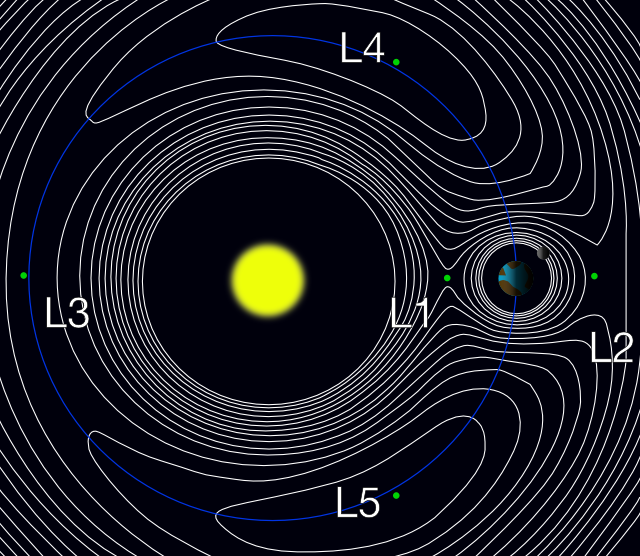The five Lagrange points of the Sun-Earth system. The white lines are gravitational contour lines: an object in free-fall would trace out a path along one of these contours.

## L1

L1 is probably the easiest Lagrange point to understand. In the diagram above the Sun pulls towards the left, and the Earth pulls to the right, and the resultant force is just right to keep it in orbit between the Sun and Earth.

The L1 point is ideal for making observations of the Sun-Earth system as satellites placed here are never in the shadow of either the Sun or the Earth. The SOHO, ACE and WIND satellites are all positioned at L1.

## L2

L2 is a good site for space-based observatories as it is almost perfectly protected from solar radiation and doesn’t receive any light pollution from Earth. The Planck telescope is currently at L2, the James Webb Space Telescope is scheduled to be placed there in 2018, and the WMAP and Herschel satellites were also previously positioned there.

## L3

No satellites are currently positioned at L3, as it lies about 300 million kilometres from Earth. It has been suggested as a useful site for solar activity monitoring, as it would be able to spot any activity likely to affect Earth (sunspots, etc.) before the side of the Sun containing that activity was pointed towards Earth.

L3 is also popular in science fiction, as a place to “hide” something from Earth. After the development of interplanetary probes we checked and unfortunately there’s nothing there (yet).

## L4 and L5

The L4 and L5 points lie at a 60° angle from the Sun-Earth axis, at the furthermost point of an equilateral triangle formed by the Sun and the Earth. Because this point is the same distance from both the Sun and Earth the ratio of their gravitational pulls is the same as if it were at the barycentre of the Sun-Earth system and objects placed there remain stationary. No satellites are currently placed at either point.

The Trojan asteroids are located at the L4 and L5 points of the Jupiter-Sun system. Asteroids are constantly being transferred between the asteroid belt and these two points.

* Lagrange points exist for any two-body system in which one object is much more massive than the other, but I’ll only be looking at the Sun-Earth system.

# The Roche limit and planetary rings

As a satellite orbits around an object (a primary), the gravitational force on the side closest to the object is greater than that on the side opposite the object. This difference in gravitational attraction gives rise to a tidal force (so-called because it is what causes the tides on Earth). As a satellite approaches closer to the body it orbits this tidal force will eventually become greater than the gravitational forces holding the satellite together. The point at which this occurs is known as the Roche limit (named for Édouard Roche who first calculated it).

The Roche limit d depends on the radius of the primary RM, the density of the primary ρM and the density of the satellite ρm.$d = 2.44\; R_M \sqrt{\frac {\rho_M} {\rho_m}}$

If we take our Earth-Moon system as an example, with the radius of Earth being 6370 kilometres, the Earth’s density as 5520 kilograms per cubic metre and the Moon’s density as 3350 kg/m3 this gives us a Roche limit of 18 400 km. The Moon’s actual orbit is 385 000 km, so luckily we don’t have to worry about the Moon breaking up any time soon.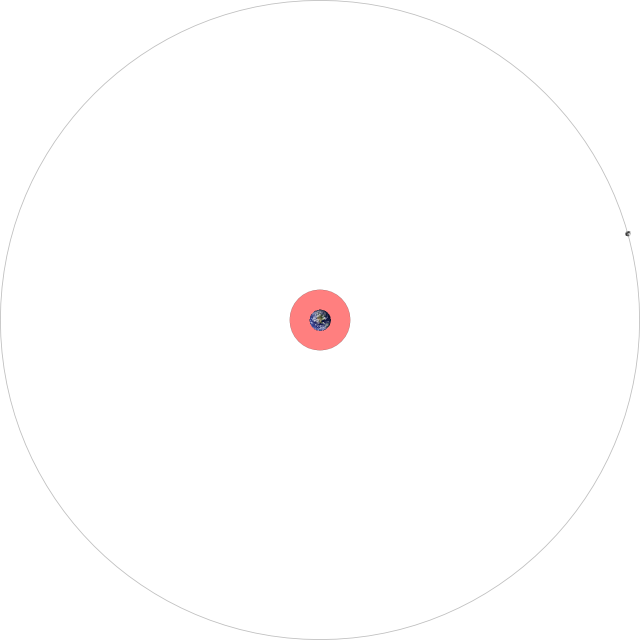The Earth-Moon system, to scale. The area beyond the Roche limit is shaded red.

When an orbiting object passes through the Roche limit it begins to break up, with the material closest to the object moving faster than the material behind it. This eventually leads to the formation of rings.